# Java Program to print Armstrong numbers between a given range

In this tutorial you will learn about the Java Program to print Armstrong numbers between a given range and its application with practical example.

In this tutorial, we will learn to create a Java Program to print Armstrong numbers between a given range using Java programming.

## Prerequisites

Before starting with this tutorial we assume that you are best aware of the following Java programming topics:

• Java Operators.
• Basic Input and Output function in Java.
• Class and Object in Java.
• Basic Java programming.
• If-else statements in Java.
• For loop in Java.

What is Armstrong number.

A number is said to  be an Armstrong number if the given number equals to the sum of the cubes of individual digits said to be Armstrong number

Example, 153 is a Armstrong Number.
153 = (1)3 + (5)3 + (3)3.
=> 153 = 1 + 125 + 27
153 = 153

## Java Program to print Armstrong numbers between a given range

In this program we would find the Armstrong number between Given Range .First of all we take range from initial point to final point form user and find the Armstrong number with in a range.let have a look at the code.

## Output

Armstrong Number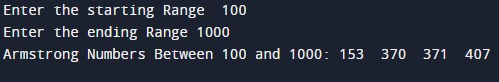When No Armstrong Number Found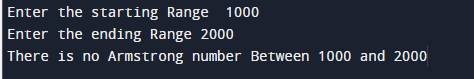In the above program, we have first declared and initialized a set variables required in the program.

• Starting = it will hold entered starting range.
• Ending = it wil hold entered last value with in the range.
• i=  for itertion.
• temp and r= it will hold temporary values.
• count=it will tell us number is Armstrong number or not.

After declaring variables we take range from user and find Armstrong number between number.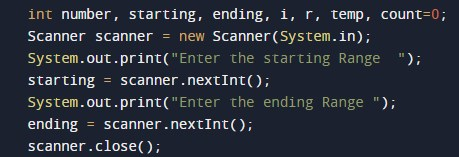## Algorithm

1.Take ranges to find Armstrong
2. Assign  values to the variables.
3. Break the digits of Armstrong numbers.
4. Then find the cubevalue of Digits.
5. then by adding all cubevalues together to check Armstrong number
6. Save the values in  variable number.
7. If number is equals to Armstrong number show Armstrong Number

all the process show in below image..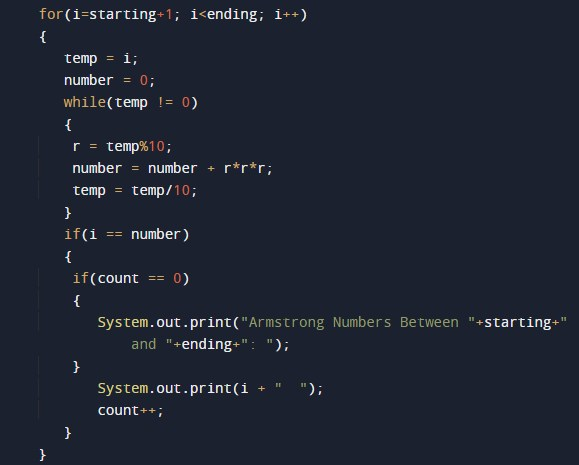if now Armstrong number found within the range print.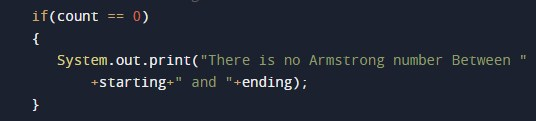In this tutorial we have learn about the Java Program to print Armstrong numbers between a given range and its application with practical example. I hope you will like this tutorial.